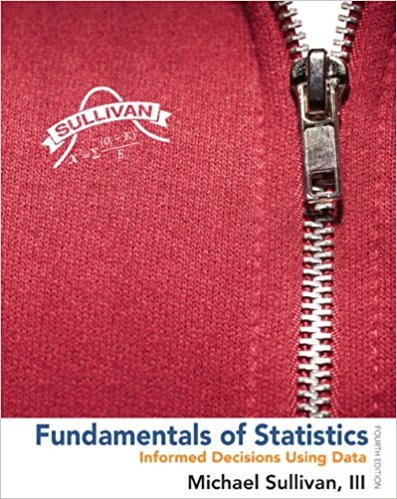×
Get Full Access to Fundamentals Of Statistics - 4 Edition - Chapter 6.2 - Problem 35ayu
Get Full Access to Fundamentals Of Statistics - 4 Edition - Chapter 6.2 - Problem 35ayu

×

# Answer: On-Time Flights According to flightstats.com,ISBN: 9780321838704 30

## Solution for problem 35AYU Chapter 6.2

Fundamentals of Statistics | 4th Edition

• Textbook Solutions
• 2901 Step-by-step solutions solved by professors and subject experts
• Get 24/7 help from StudySoup virtual teaching assistantsFundamentals of Statistics | 4th Edition

4 5 1 388 Reviews
29
3
Problem 35AYU

On-Time Flights According to flightstats.com, American Airlines flights from Dallas to Chicago are on time 80% of the time. Suppose 15 flights are randomly selected, and the number of on-time flights is recorded.

(a) Explain why this is a binomial experiment.

(b) Find and interpret the probability that exactly 10 flights are on time.

(c) Find and interpret the probability that fewer than 10 flights are on time.

(d) Find and interpret the probability that at least 10 flights are on time.

(e) Find and interpret the probability that between 8 and 10 flights, inclusive, are on time.

Step-by-Step Solution:
Step 1 of 2

Step1 of 2

We have According to flightstats.com, American Airlines flights from Dallas to Chicago are on time 80% of the time. Suppose 15 flights are randomly selected, and the number of on-time flights is recorded.

a).

This is a binomial experiment because:

1.  It is performed a fixed number of times, n = 15.

2.  The trials are independent.

3.  For each trial, there are two possible mutually exclusive outcomes: on time and not on time.

4.  The probability of “on time” is fixed at p = 0.80.

b).

We have XB(15, 0.80).

Probability mass function of binomial distribution is given by

P(X) =, x = 0,1,2,.....,15.

We need to find P(3) = ?.

Consider,

P(X)  =, x = 0,1,2,.....,15.

P(10)   ==  3003*(0.1073)*(0.00032)

= 0.1031110.1032.

Therefore,the probability of x successes in the n independent trials of the experiment is P(38) = 0.1032.

In 100 trials of this experiment, we expect about 10 trials to result in exactly 10 flights being on time.

c).

The probability that fewer than 10 flights are on time is given by P(X < 10)

Probability mass function of binomial distribution is given by

P(X) =, x = 0,1,2,.....,15.

P(X) =, x = 0,1,2,.....,15.

Put x = 0,1,2,.....,9. In above equation we get  P(X < 10)

 x P(X) 0 3.2768E-11 1 1.9661E-09 2 5.505E-08 3 9.542E-07 4 1.145E-05 5 0.00010076 6 0.00067176 7 0.00345476 8 0.01381906 9 0.04299262 P(X < 10 ) = 0.06105143

Therefore,The probability that fewer than 10 flights are on time is P(X < 10 ) = 0.06105143.

In 100 trials of this experiment, we expect about 6 trials to result in fewer than 10 flights being on time.

____________

Step 2 of 2

##### ISBN: 9780321838704

Unlock Textbook Solution